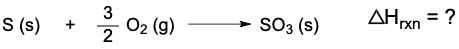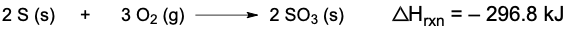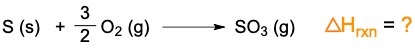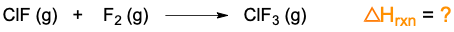Clutch Prep is now a part of Pearson
Ch.7 Energy, Rate and EquilibriumWorksheetSee all chapters

# Hess's Law

See all sections
Sections
Nature of Energy
First Law of Thermodynamics
Endothermic & Exothermic Reactions
Bond Energy
Thermochemical Equations
Heat Capacity
Thermal Equilibrium (Simplified)
Hess's Law
Rate of Reaction
Energy Diagrams
Chemical Equilibrium
The Equilibrium Constant
Le Chatelier's Principle
Solubility Product Constant (Ksp)
Spontaneous Reaction
Entropy (Simplified)
Gibbs Free Energy (Simplified)

Hess's Law involves the use of partial reactions in the determination of the overall enthalpy of reaction.

###### Hess's Law

Concept #1: Rearrangement of Thermochemical Equations

Example #1: If the formation equation for boron trioxide is given as the following:

What will be the new enthalpy value when it is rearranged?

Practice: Calculate the ∆Hrxn for the following thermochemical equation:When given the following:Concept #2: Applying Hess’s Law

Example #2: Calculate the ∆Hrxn for overall reaction:

When given the following set of partial reactions:

Practice: Calculate the ∆Hrxn forGiven the following set of reactions:Practice: Calculate the ∆Hrxn forGiven the following reactions: